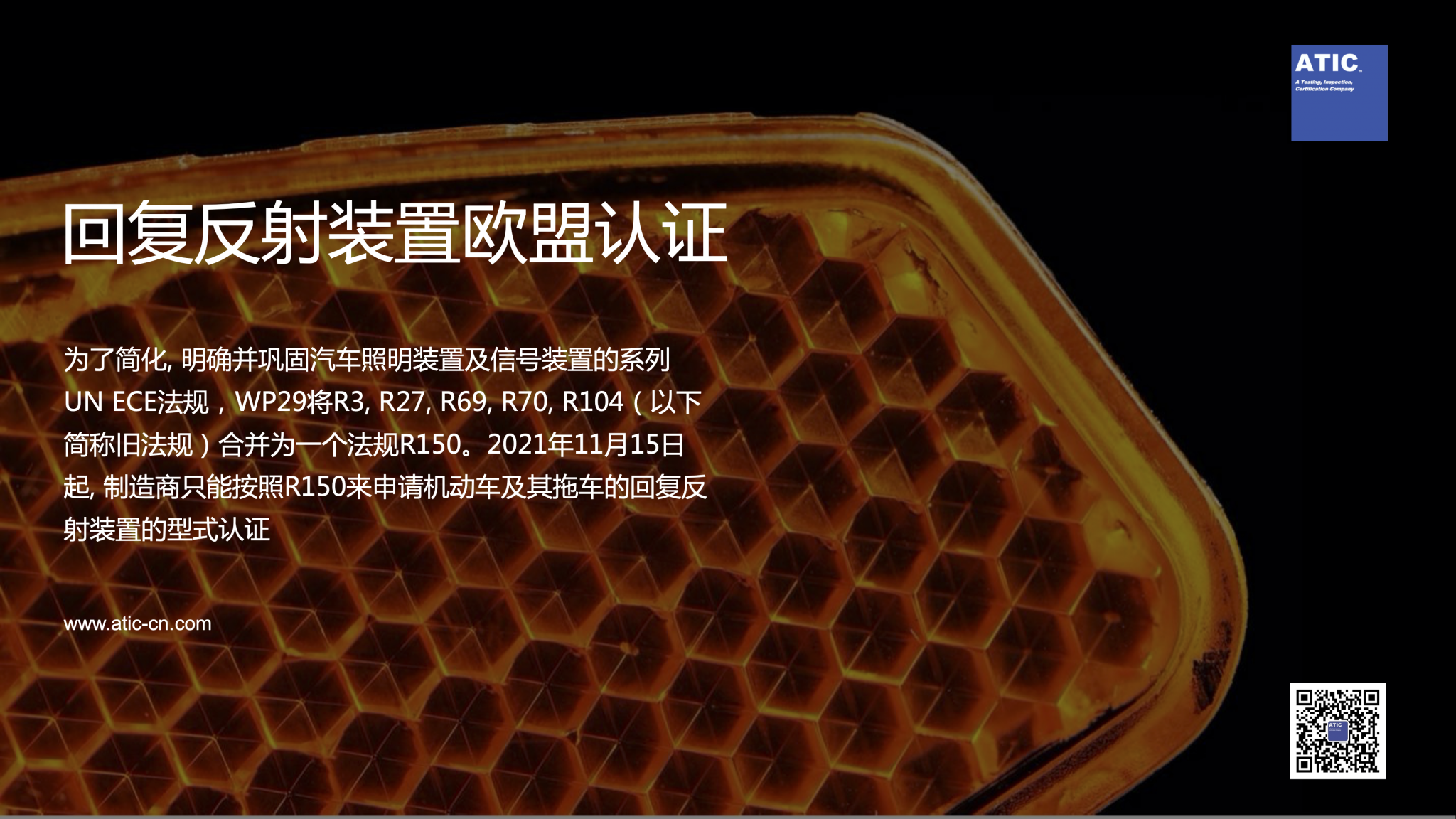# 【泰测 | 认证知识】UN R150回复反射装置欧盟认证解析2021年11月15日起：
• 适用R150的缔约国应停止批准关于旧版法规的新申请；
• 已批准的旧版法规证书不会因此失效，如需进行证书延伸，持证的制造商可按照旧法规及其修正案申请；
• 如果是用于替换目的后装件，也可以按照旧法规申请。

R150认证范围
• 回复反射器： IA级,  IB级,  IIIA级,  IIIB级,  IVA级
• 回复反射标志： C级,  D级,  E级,  F级
• 重型和长型车辆的回复反射标志板：1类, 2类, 3类, 4类, 5类
• 低速行驶车辆的回复反射标志板：1类和2类
• 三角警告牌：1类和2类

• 资料准备，提出申请
• 送样测试
• 工厂审核（持有效体系证书可免去审厂）
• 提交资料
• 颁发证书
R150的认证流程, 周期与旧版法规基本一致，个别交通部有COP扩项要求，详情请咨询泰测。

 回复反射器 回复反射标记 回复反射标志板 三角警告牌 IA，IB IIIA, IIIB IVA C D，E F 重型长型车 SMV 1, 2, 3, 4 5 1, 2 1, 2 形状及尺寸 X X X X X X X X X X 光度 X X X X X X X X X X 色度 X X X X X X X X X 反射系数 X X X X X X X X X 耐热 X X X X X X X X X 水渗透 X X X X A X X X X X 对燃料的耐受性 X X X A X 对润滑剂耐受性 X X X X X X X X 耐腐蚀 X X X 后表面的磨蚀强度试验 X X X X X X 耐候性 X X X X X X X X 光学性能的时间稳定性测试 X X X X 耐清洗 X X X X X 粘合强度 A A A A A 弯曲度 X 冲击 A A A A A 板材刚性 X X X 色牢度 X 对地间隙机械稳固性高低温抗风稳定性 X X X X X X X X X X
*X=A=如需Alex / 贺润润

Phone: +86 15518506723
Mail: alex.he@atic-cn.com